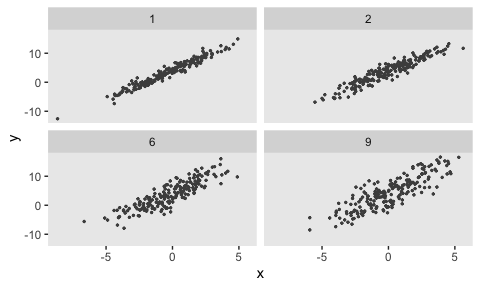# Dynamic Data Definition

Often, we’d like to explore data generation and modeling under different scenarios. For example, we might want to understand the operating characteristics of a model given different variance or other parametric assumptions. There is functionality built into simstudy to facilitate this type of dynamic exploration. First, there are functions updateDef and updateDefAdd that essentially allow one to edit lines of existing data definition tables. Second, there is a built in mechanism - called double-dot reference - to access external variables that do not exist in a defined data set or data definition.

### Updating existing definition tables

The updateDef function updates a row in a definition table created by functions defData or defRead. Analogously, updateDefAdd function updates a row in a definition table created by functions defDataAdd or defReadAdd.

The original data set definition includes three variables x, y, and z, all normally distributed:

defs <- defData(varname = "x", formula = 0, variance = 3, dist = "normal")
defs <- defData(defs, varname = "y", formula = "2 + 3*x", variance = 1, dist = "normal")
defs <- defData(defs, varname = "z", formula = "4 + 3*x - 2*y", variance = 1, dist = "normal")

defs
##    varname       formula variance   dist     link
## 1:       x             0        3 normal identity
## 2:       y       2 + 3*x        1 normal identity
## 3:       z 4 + 3*x - 2*y        1 normal identity

In the first case, we are changing the relationship of y with x as well as the variance:

defs <- updateDef(dtDefs = defs, changevar = "y", newformula = "x + 5", newvariance = 2)
defs
##    varname       formula variance   dist     link
## 1:       x             0        3 normal identity
## 2:       y         x + 5        2 normal identity
## 3:       z 4 + 3*x - 2*y        1 normal identity

In this second case, we are changing the distribution of z to Poisson and updating the link function to log:

defs <- updateDef(dtDefs = defs, changevar = "z", newdist = "poisson", newlink = "log")
defs
##    varname       formula variance    dist     link
## 1:       x             0        3  normal identity
## 2:       y         x + 5        2  normal identity
## 3:       z 4 + 3*x - 2*y        1 poisson      log

And in the last case, we remove a variable from a data set definition. Note in the case of a definition created by defData that it is not possible to remove a variable that is a predictor of a subsequent variable, such as x or y in this case.

defs <- updateDef(dtDefs = defs, changevar = "z", remove = TRUE)
defs
##    varname formula variance   dist     link
## 1:       x       0        3 normal identity
## 2:       y   x + 5        2 normal identity

### Double-dot external variable reference

For a truly dynamic data definition process, simstudy (as of version 0.2.0) allows users to reference variables that exist outside of data generation. These can be thought of as a type of hyperparameter of the data generation process. The reference is made directly in the formula itself, using a double-dot (“..”) notation before the variable name. Here is a simple example:

def <- defData(varname = "x", formula = 0,
variance = 5, dist = "normal")
def <- defData(def, varname = "y", formula = "..B0 + ..B1 * x",
variance = "..sigma2", dist = "normal")

def
##    varname         formula variance   dist     link
## 1:       x               0        5 normal identity
## 2:       y ..B0 + ..B1 * x ..sigma2 normal identity
B0 <- 4;
B1 <- 2;
sigma2 <- 9

set.seed(716251)
dd <- genData(100, def)

fit <- summary(lm(y ~ x, data = dd))

coef(fit)
##             Estimate Std. Error  t value     Pr(>|t|)
## (Intercept)  4.00368  0.2839423 14.10033 2.559075e-25
## x            2.01001  0.1303472 15.42043 5.904268e-28
fit$sigma ##  2.827271 It is easy to create a new data set on the fly with a difference variance assumption without having to go to the trouble of updating the data definitions. sigma2 <- 16 dd <- genData(100, def) fit <- summary(lm(y ~ x, data = dd)) coef(fit) ## Estimate Std. Error t value Pr(>|t|) ## (Intercept) 4.351536 0.4268310 10.194987 4.569364e-17 ## x 2.123973 0.2179406 9.745651 4.315348e-16 fit$sigma
##  4.210897

The double-dot notation can be flexibly applied using lapply (or the parallel version mclapply) to create a range of data sets under different assumptions:

sigma2s <- c(1, 2, 6, 9)

gen_data <- function(sigma2, d) {
dd <- genData(200, d)
dd\$sigma2 <- sigma2
dd
}

dd_4 <- lapply(sigma2s, function(s) gen_data(s, def))
dd_4 <- rbindlist(dd_4)

ggplot(data = dd_4, aes(x = x, y = y)) +
geom_point(size = .5, color = "grey30") +
facet_wrap(sigma2 ~ .) +
theme(panel.grid = element_blank())The double-dot notation is also array-friendly. For example if we want to create a mixture distribution from a vector of values (which we can also do using a categorical distribution), we can define the mixture formula in terms of the vector. In this case we are generating permuted block sizes of 2 and 4:

defblk <- defData(varname = "blksize",
formula = "..sizes | .5 + ..sizes | .5", dist = "mixture")

defblk
##    varname                           formula variance    dist     link
## 1: blksize ..sizes | .5 + ..sizes | .5        0 mixture identity
sizes <- c(2, 4)
genData(1000, defblk)
##         id blksize
##    1:    1       4
##    2:    2       4
##    3:    3       4
##    4:    4       2
##    5:    5       4
##   ---
##  996:  996       2
##  997:  997       2
##  998:  998       4
##  999:  999       4
## 1000: 1000       4